# Trefftz method

(diff) ← Older revision | Latest revision (diff) | Newer revision → (diff)

One of the variational methods for solving boundary value problems. Suppose one has to solve the boundary value problem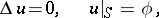(*)

whereis the boundary of a domain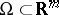. The solution of the problem (*) minimizes the functionalover all functions satisfying the boundary condition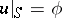. Trefftz' method consists in the following. Suppose one is given a sequence of harmonic functionsinthat are square summable intogether with their first derivatives. An approximate solution is sought in the formthe coefficientsbeing determined from the condition that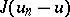is minimal, whereis the exact solution of (*). This leads to the following system of equations for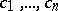: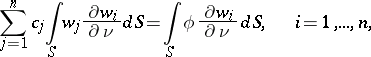whereis the outward normal to.

Trefftz' method can be generalized to various boundary value problems (see ).

The method was proposed by E. Trefftz (see ).

How to Cite This Entry:
Trefftz method. Encyclopedia of Mathematics. URL: http://encyclopediaofmath.org/index.php?title=Trefftz_method&oldid=12688
This article was adapted from an original article by G.M. Vainikko (originator), which appeared in Encyclopedia of Mathematics - ISBN 1402006098. See original article